Latest Banking jobs   »

# Quantitative Aptitude Quiz For IBPS RRB PO Prelims 2022- 10th June

Directions (1-5): Given bar graph shows total number of confirmed cases of COVIND-19 and number of deaths in four different countries. Study the bar graph carefully and answer the questions given below.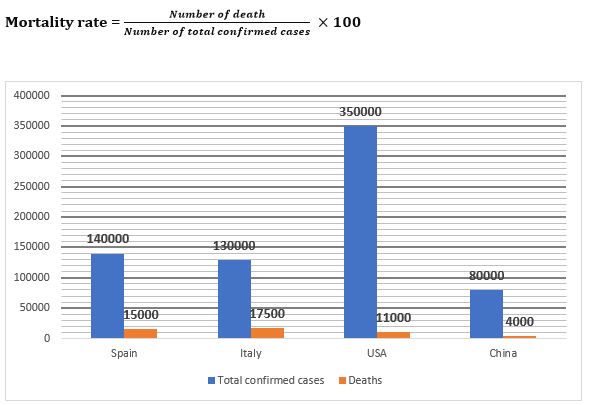Q1. For which country mortality rate is lowest among the given four countries.
(a) Italy
(b) USA
(c) Spain
(d) China
(e) USA and China

Q2. Total confirmed cases in USA is what percent more than total deaths in Italy.
(a) 1200%
(b) 1350%
(c) 2100%
(d) 1900%
(e) 1500%

Q3. Find out the ratio between mortality rate of Spain to that of China?
(a) 19: 11
(b) 43:14
(c) 15:7
(d) 14:9
(e) 13: 5

Q4. Total death in all four countries together is what percent of total confirmed cases in China?
(a) 59.375%
(b) 62%
(c) 55%
(d) 66.66%
(e) 75%

Q5. If number of confirmed cases in China is increased by 25% and mortality rate remains same, what will be the new number of total deaths in China.
(a) 4400
(b) 4500
(c) 4600
(d) 5200
(e) 5000

Directions (6 – 10): Given below the bar graph shows the quantity of six different items (in kg) purchased by a person during the lockdown period. Read the data carefully and answer the questions.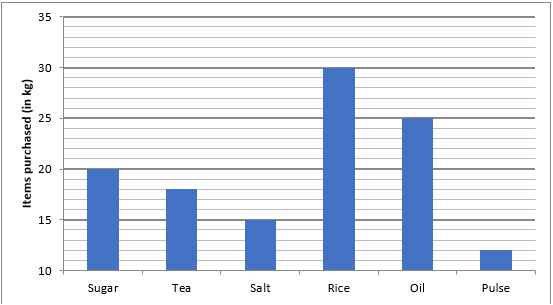Q6. If the sum of the price of one kg sugar and one kg salt is Rs.84 and the ratio of price of one kg of sugar and one kg of salt is 11: 10. Then, find the difference between the total price of Sugar and salt purchased by man?
(a) Rs. 220
(b) Rs. 240
(c) Rs. 260
(d) Rs. 300
(e) Rs. 280

Q7. If the total price of tea is Rs. 900 and that of rice is Rs. 1500, then find the price of one kg tea is what percent more than that of rice?
(a) 0%
(b) 20%
(c) 5%
(d) 10%
(e) 15%

Q8. If the price of one kg of pulse and one kg of oil is Rs. 63 and Rs. 42 respectively, then find the ratio of the total price of the pulse to the total price of oil?
(a) 13:25
(b) 1:2
(c) 3:5
(d) 18:25
(e) 12:13

Q9. The total quantity of sugar and salt purchased together by man is what percent of the total quantity of rice and pulse together purchased by man?
(a) 87 ⅓%
(b) 83 ⅓%
(c) 74%
(d) 92%
(e) 64 ⅓%

Q10. If the price of one kg salt, one kg rice, and one kg oil is Rs. 56, Rs. 32 and Rs. 40 respectively, then find out the total price of oil, salt, and rice purchased by man?
(a) Rs. 2000
(b) Rs. 2800
(c) Rs. 2200
(d) Rs. 1800
(e) Rs. 2600

Directions (11-15): Study the following bar graph and answer the questions that follow.
Given below is the bar graph which shows the number of students playing three different games in five colleges in year 2014.
NOTE- one student plays only one sport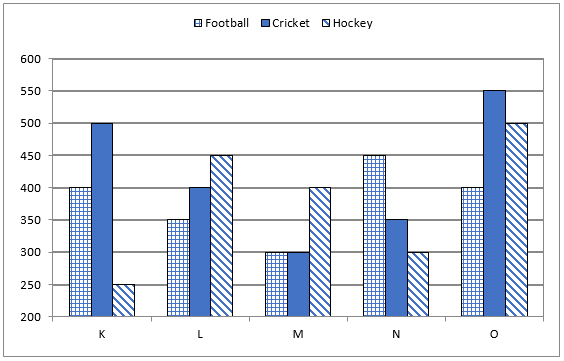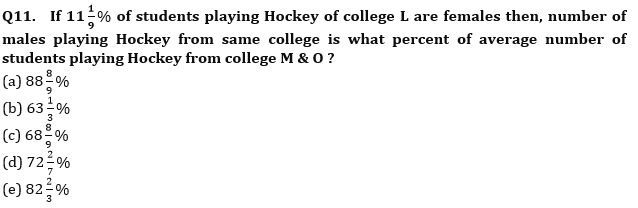Q12. If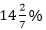of student playing Cricket of college N left playing cricket and started playing Football in same college then find the ratio of number of student playing football of college N and M together to the number of student playing Cricket of college K and N together?
(a) 3 : 2
(b) 1 : 2
(c) 1 : 1
(d) 1 : 3
(e) 2 : 1

Q13. Average no. of students playing Hockey of college K, L and O is how much more than average number of students playing football of college K, L & M ?
(a) 120
(b) 50
(c) 80
(d) 40
(e) 100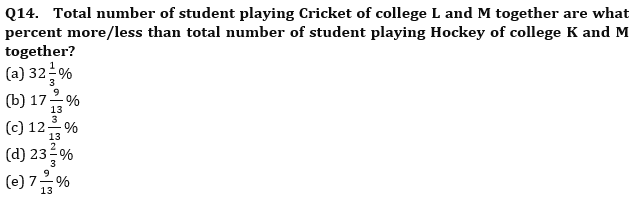Q15. If total number of students in college K in year 2015 is increased by 20% percent with respect to year 2014 and the ratio of student playing Football, Cricket and Hockey becomes 5 : 2 : 3 respectively then find the average number of students playing football in same college K in year 2014 and 2015 ?
(a) 640
(b) 525
(c) 625
(d) 545
(e) 454

Solutions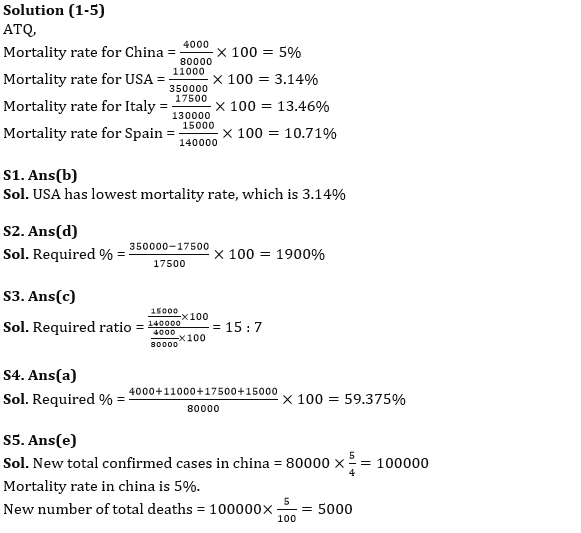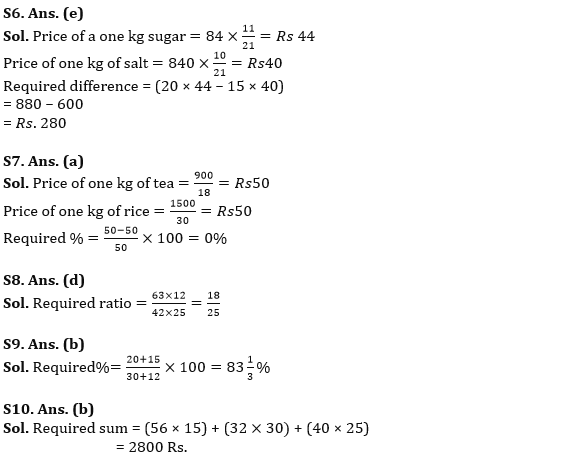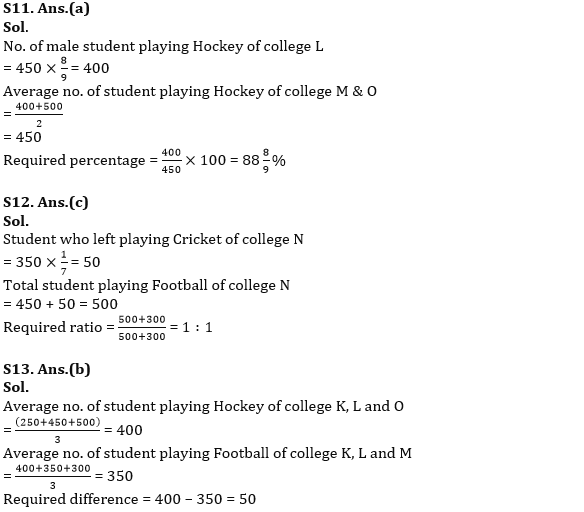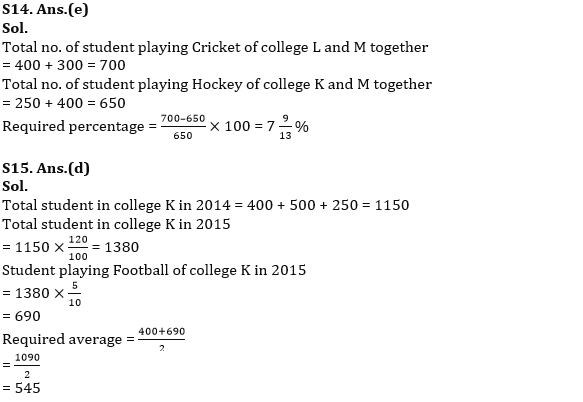#### Congratulations!